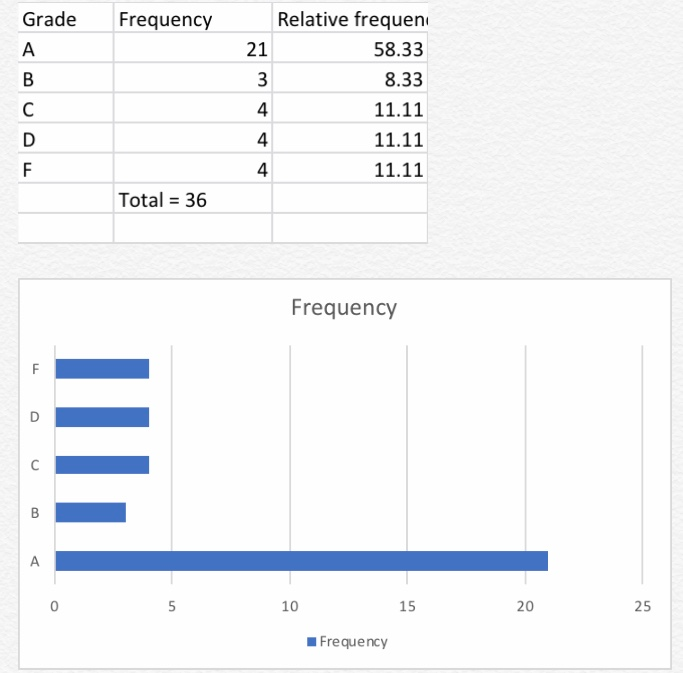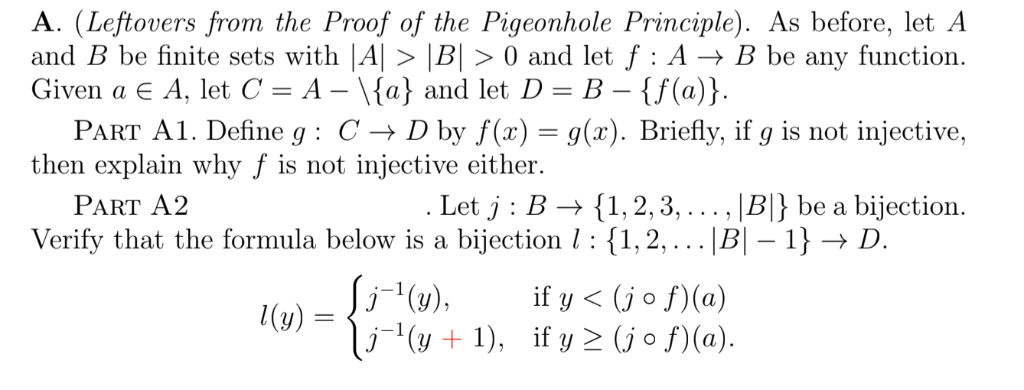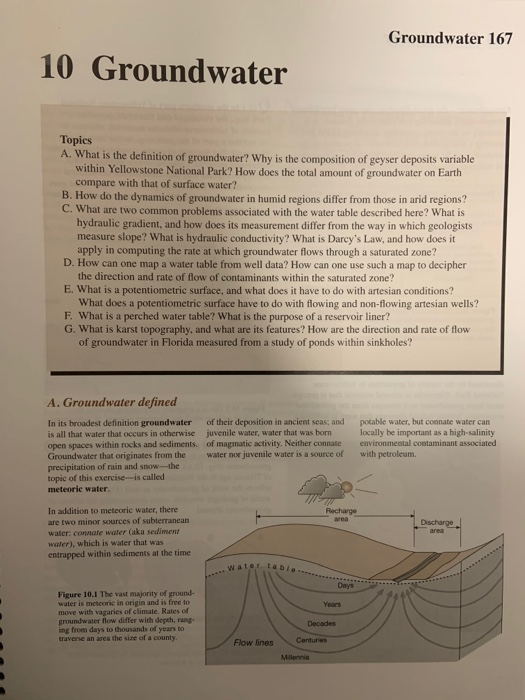Question

# Water quality ratings (A, B, C, D, F) which reflect the risk of getting sick from...

Water quality ratings (A, B, C, D, F) which reflect the risk of getting sick from swimming at any particular one of 36 Southern California beaches, are given in the following list: A A A F A A A B A C C A A F B A C A D F A D A A D A A D A A A A C F B A (a) (4 marks). Summarize the given ratings by constructing a relative frequency distribution and a bar chart. (b) (2 marks). Assuming that an A means little risk of getting sick, does it appear that the majority of beaches in Southern California are safe? On what information do you base your answer? (c) (2 marks). Would it be appropriate to construct a dotplot for these data? Why or why not?

a) We calculate relative frequency using the formula = frequency/ sum of all frequencies *100b) Since the relative frequency of A which implies little risk of getting sick is quite high i.e 58.33% it means most of the population is safe. The people with dangers of getting sick are lesser and the majority ie more than 50% belong to rating A and thus are safe.

c)A dot plot can be used here since there are only 5 categories which can be easily represented and the corresponding frequency can be plotted there on.

#### Earn Coins

Coins can be redeemed for fabulous gifts.

Similar Homework Help Questions
• ### Water quality ratings (A, B, C, D, F) which reflect the risk of getting sick from swimming at any particular one of 36 Southern California beaches, are given in the following list: A A A F A A A B A C...

Water quality ratings (A, B, C, D, F) which reflect the risk of getting sick from swimming at any particular one of 36 Southern California beaches, are given in the following list: A A A F A A A B A C C A A F B A C A D F A D A A D A A D A A A A C F B A (a) (4 marks). Summarize the given ratings by constructing a relative frequency...

• ### A. (Leftovers from the Proof of the Pigeonhole Principle). As before, let A and B be finite sets with A! 〉 BI 〉 0 and let f : A → B be any function Given a A. let C-A-Va) and let D-B-{ f(a)} PaRT...A. (Leftovers from the Proof of the Pigeonhole Principle). As before, let A and B be finite sets with A! 〉 BI 〉 0 and let f : A → B be any function Given a A. let C-A-Va) and let D-B-{ f(a)} PaRT A1. Define g: C -> D by f(x)-g(x). Briefly, if g is not injective, then explain why f is not injective either. Let j : B → { 1, 2, 3, . . . , BI}...

• ### Word Bank a. Arbitration b. Plaintiff c. Risk Management d. Negligence Tort e Respondent Superior f....

Word Bank a. Arbitration b. Plaintiff c. Risk Management d. Negligence Tort e Respondent Superior f. Deposition g. Statute of Limitations h. Defendant i. Patient Incompetence j. Durable Power of Attorney k. Uniform Anatomical Gift Act l. Risk Management m. Locum tenens n. Res ipsa loquitor o. Informed Consent p. Implied Consent q. Living will r. Emancipated minor s. Expressed consent t. Mediation approach u. Subpoena duces tecum v. Good Samaritan Law w. Malpractice x. Patient Self Determination Act y....

• ### please answer all the questions written in brown. Groundwater 167 10 Groundwater Topics A. What is...please answer all the questions written in brown. Groundwater 167 10 Groundwater Topics A. What is the definition of groundwater? Why is the composition of geyser deposits variable within Yellowstone National Park? How does the total amount of groundwater on Earth compare with that of surface water? B. How do the dynamics of groundwater in humid regions differ from those in arid regions? C. What are two common problems associated with the water table described here? What is hydraulic gradient,...